# Get N random Rows from a NumPy Array in PythonLast updated: Aug 3, 2023
3 min## #Get N random Rows from a NumPy Array in Python

To get N random rows from a NumPy array:

1. Use the `numpy.random.randint()` method to get an array of random indexes.
2. Use bracket notation to select the random rows with the indexes array.
main.py
```Copied!```import numpy as np

arr = np.array([
[2, 4, 6],
[1, 3, 5],
[3, 5, 7],
[4, 6, 8],
[5, 7, 9]
])

index = np.random.randint(arr.shape, size=2)

print(index)  # 👉️ [3 2]

print('-' * 50)

random_rows = arr[index, :]

# [[4 6 8]
#  [3 5 7]]
print(random_rows)
``````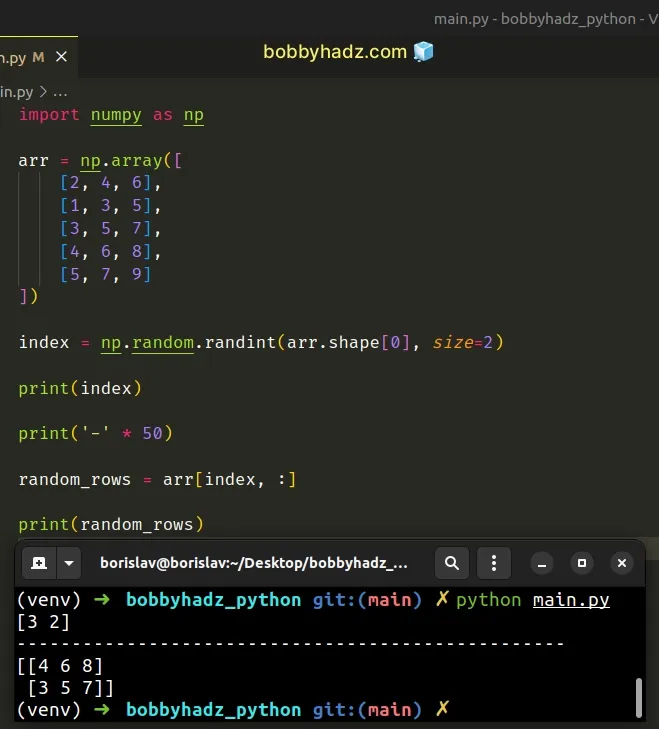The numpy.random.randint method returns an array of random integers that we can use to index the original NumPy array.

The code sample selects 2 random rows from the NumPy array with replacement.

In other words, rows can be repeated.

Notice that in the following example, the array of random indexes has repeat values.

main.py
```Copied!```import numpy as np

arr = np.array([
[2, 4, 6],
[1, 3, 5],
[3, 5, 7],
[4, 6, 8],
[5, 7, 9]
])

index = np.random.randint(arr.shape, size=4)

print(index)  # 👉️ [1 2 1 4]

print('-' * 50)

random_rows = arr[index, :]

# [[1 3 5]
#  [3 5 7]
#  [1 3 5]
#  [5 7 9]]
print(random_rows)
``````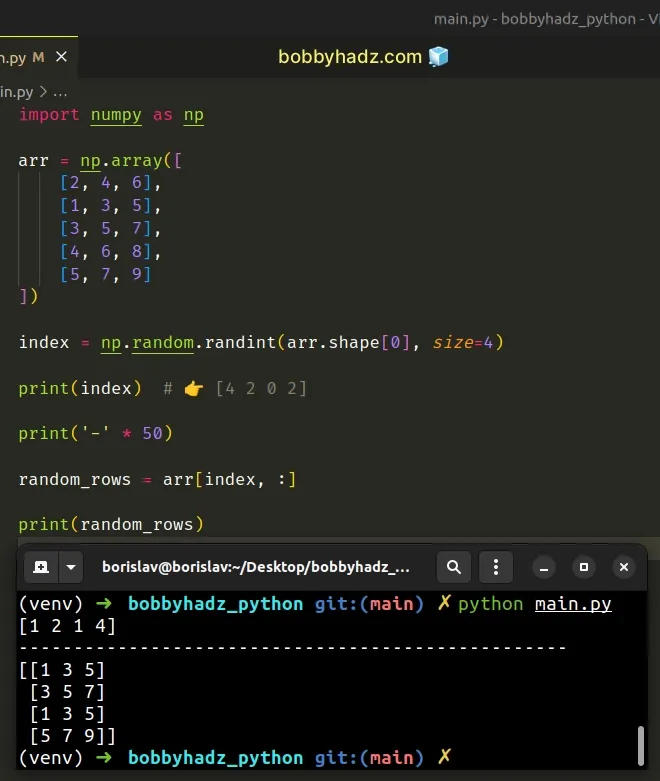The code sample selects 4 random rows from the NumPy array with replacement (with repeats).

## #Get N random Rows from a NumPy Array without replacement

If you need to get N random rows from a NumPy array without replacement (without duplicates), use the `numpy.random.choice()` method instead.

main.py
```Copied!```import numpy as np

arr = np.array([
[2, 4, 6],
[1, 3, 5],
[3, 5, 7],
[4, 6, 8],
[5, 7, 9]
])

index = np.random.choice(
arr.shape,
2,
replace=False
)

print(index)  # 👉️ [1 0]

print('-' * 50)

random_rows = arr[index, :]

# [[1 3 5]
#  [2 4 6]]
print(random_rows)
``````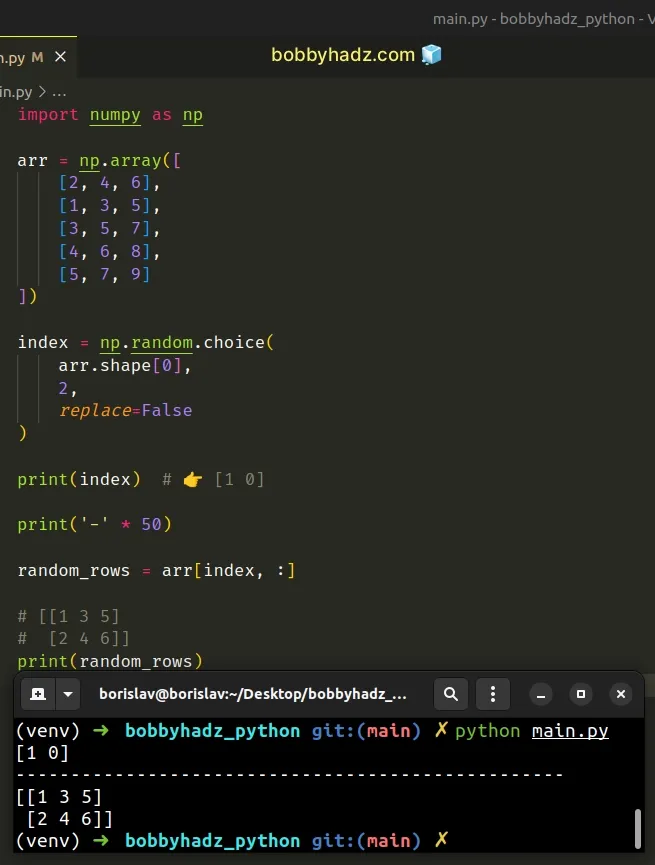The numpy.random.choice method generates a random sample from a given 1-D array.

We used the method to generate an array of 2 random indexes.

The `replace` argument determines whether the generated random sample is with or without replacement.

We set the argument to `False` to generate the sample without replacement.

By default, it is set to `True`.

You won't get duplicate indexes (and rows) when `replace` is set to `False`.

## #Defining a reusable function

If you have to do this often, define a reusable function.

main.py
```Copied!```import numpy as np

def random_rows(array, size=1):
return array[
np.random.choice(len(array), size=size, replace=False),
:
]

arr = np.array([
[2, 4, 6],
[1, 3, 5],
[3, 5, 7],
[4, 6, 8],
[5, 7, 9]
])

print(random_rows(arr, 2))

print('-' * 50)

print(random_rows(arr, 3))
``````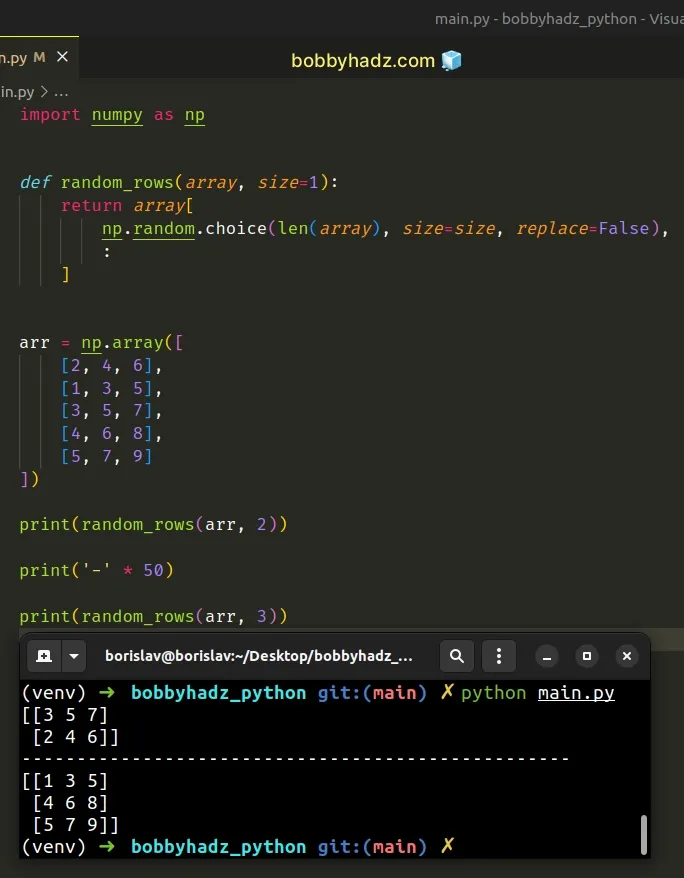The function takes a NumPy array and the number of random rows as parameters.

It uses the `numpy.random.choice()` method to get an array of random indexes and accesses the supplied array at the generated indexes.

The `replace` argument is set to `False`, so no duplicate rows will be selected.

## #Get N random Rows from a NumPy Array using `numpy.random.shuffle()`

You can also use the numpy.random.shuffle method to get N random rows from a NumPy array.

main.py
```Copied!```import numpy as np

arr = np.array([
[2, 4, 6],
[1, 3, 5],
[3, 5, 7],
[4, 6, 8],
[5, 7, 9]
])

np.random.shuffle(arr)

# [[4 6 8]
#  [5 7 9]]
print(arr[:2, :])

print('-' * 50)

# [[4 6 8]
#  [5 7 9]
#  [2 4 6]]
print(arr[:3, :])
``````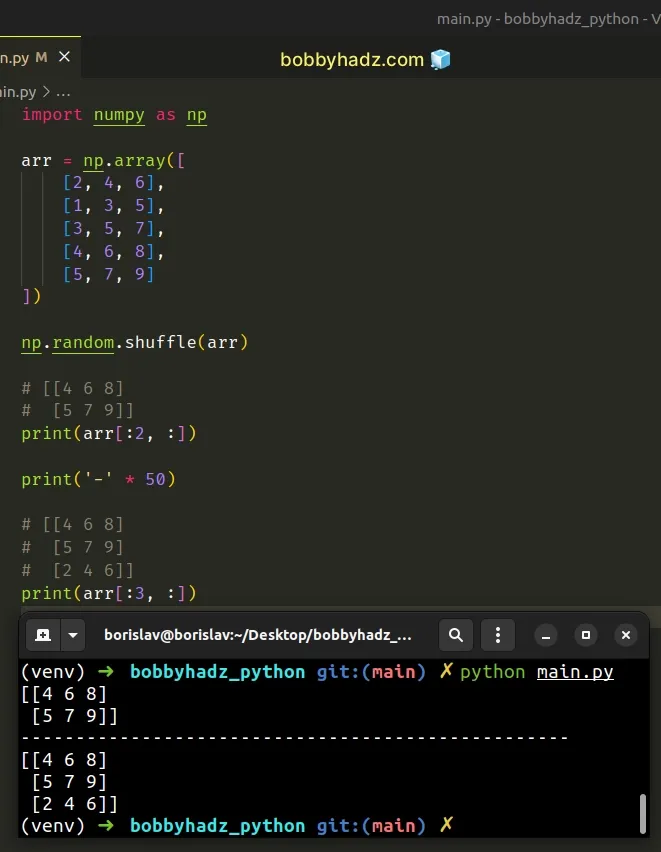The `numpy.random.shuffle` method shuffles the NumPy array in place and returns `None`.

main.py
```Copied!```import numpy as np

arr = np.array([
[2, 4, 6],
[1, 3, 5],
[3, 5, 7],
[4, 6, 8],
[5, 7, 9]
])

np.random.shuffle(arr)

# [[1 3 5]
#  [4 6 8]
#  [5 7 9]
#  [3 5 7]
#  [2 4 6]]
print(arr)
``````

Once we have the shuffled array, we can use indexing to select N random rows.

I've also written an article on how to shuffle two NumPy arrays in unison.